# RD Sharma Solutions Chapter 8 Quadratic Equations Exercise 8.13 Class 10 Maths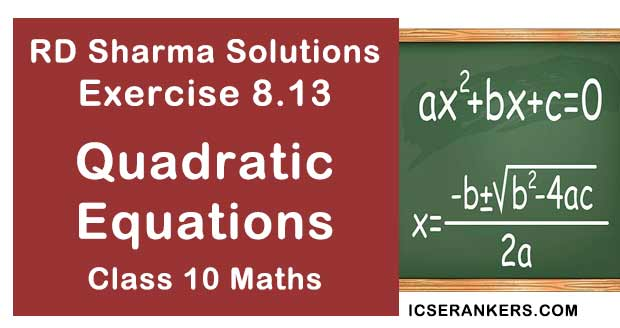Chapter Name RD Sharma Chapter 8 Quadratic Equations Book Name RD Sharma Mathematics for Class 10 Other Exercises Exercise 8.1Exercise 8.2Exercise 8.3Exercise 8.4Exercise 8.5Exercise 8.6Exercise 8.7Exercise 8.8Exercise 8.9Exercise 8.10Exercise 8.11Exercise 8.12 Related Study NCERT Solutions for Class 10 Maths

### Exercise 8.13 Solutions

1. A piece of cloth costs Rs. 35. If the piece were 4m longer and each metre costs Rs. one less, the cost would remain unchanged. How long is the piece ?

Solution

Let initial length of the cloth be x m, and cost per each meter of cloth b Rs y
⇒ Total cost of piece of cloth will be length of cloth x cost per each meter = xy
But given that xy = Rs. 35 ⇒ y = Rs. 35/x
And also,
Given that if the piece were 4m longer and each meter costs Rs. 1 less the cost would remain unchanged.
⇒ Length of the cloth will be (x + 4)m and cost per each meter of cloth will be Rs(y..)
⇒ Total cost of piece of cloth will be Rs. (x + 4)(y - 1)
But,
Rs (x + 4)(y - 1) = Rs 35
⇒ xy + 4y -x - 4 = 35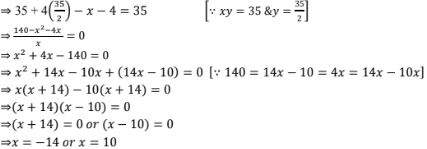Since length of the cloth cannot be in negative integers, the required length of cloth is 10 m.

2. Some students planned a picnic. The budget for food was Rs. 480. But eight of these failed to go and thus the cost of food for each member increased by Rs. 10. How many students attended the picnic ?

Solution

Let x be no. of students planned for a picnic
Given that budget for food was Rs 480
⇒ Share of each student = (Total budget)/(no. of students) = Rs. 480/x
Given that 8 students foiled to go
⇒ No. of students will be (x - 8)
Now,
share of each student will be equal to = (total budget)/(No. of students) = Rs. 480/(x - 8)
Given that if 8 students failed to go, then cost of food for each member increased by Rs. 10.

3. A dealer sells an article for Rs. 24 and gains as much percent as the cost price of the article. Find the cost price of the article.

Solution

Let the cost price of the article be Rs x
Given that gain percentage of the article is as much as cost price i.e. x
⇒ Selling price = cost  + price + gain
= Rs x  + cost price × gain percentage
= Rs x + Rs x × x/100
= Rs (x + x2 /100)
Given that selling price = Rs 24
⇒ Rs 24 = Rs (x + x2 /100)
⇒ 24 = x + x2 /100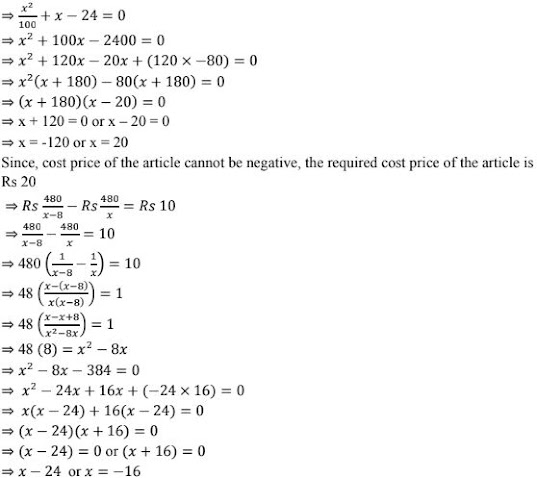Since the value of number of students cannot be negative, the required number of students attended the picnic is 24.

4. Out of a group of swans, 7/2 times the square root of the total number are playing on he share of a pond. The two remaining ones are swinging in water. Find the total number of swans.

Solution

Let total number of swans be x
Given that 7/2 times the square root of the total number of swans are playing on the share of a pond i.e. (7/2)√x and the two remaining ones are swinging in water.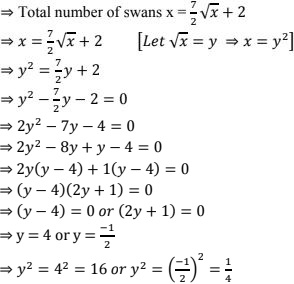Since, the value of number of swans cannot be a fraction, the required number of swans x = 16

5. If the list price of a toy is reduced by Rs. 2, a person can buy 2 toys more for Rs. 360. Find the original price of the toy.

Solution

Let initial list price of the toy be Rs x
Given that total cost of toys = Rs 360
⇒ Initially number of toys a person can buy  = (Total cost)/(list price of each toy) = Rs. 360/Rs (x -2) = 360/(x-2)
Now,6. Rs 9000 were divided equally among a certain number of persons. Had there been 20 more persons, each would have got Rs. 160 less. Find the original number of persons.

Solution

Let the original number of persons be x.
Total amount to be divided equally is Rs. 9000
⇒ Share of each person will be equal to (total amount)/(No.of persons) = Rs. 900/x
Given that if there had been 20 more persons
⇒ Final number of persons will be x + 20, then each would have got Rs 160 less
Now,
Final share of each person will be equal to = (total amount)/(No. of persons) = Rs 9000/(x + 20)
We have,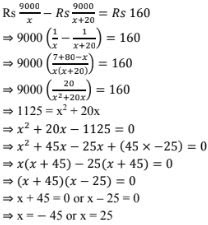Since, share of each person cannot be negative value, the required share of each person is Rs. 25.

7. Some students planned a picnic. The budget for food was Rs. 500. But, 5 of them failed to go and thus the cost of food for each member increased by Rs. 5. How many students attended the picnic ?

Solution

Let the number of students planned for the picnic be x
Given budget for food = Rs 500
⇒ Initially share of food for each student = (total budget)/(no. of students) = Rs. 500/x
given that 5 students failed to go for the picnic
⇒ No. of students attended the picnic will be (x - 5)
Now, share of food for each student will be equal to = (total budget)/(no. of students attended) = Rs. 500/(x - 5)
Given that, share of food for each student is increased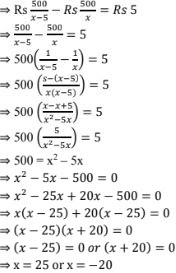Since, the value of x cannot be negative,
⇒ x = 25
Here, x is the no. of students planned,
Given that 5 students failed to go
⇒ No. of students attended the picnic = x - 5 =25 - 5 = 20
∴ No. of students attended the picnic = 20

8. A pole has to erected at a point on the boundary of a circular park of diameter 13 metres in such a way  that the difference of its distances from two diametrically opposite fixed gates A and B on the boundary is 7 metres. Is it the possible to do so ? If yes at what distance from the two gates should the pole be erected ?

Solution

Let P be the required location of the pole such that its distance from gets B is x meters i.e.
BP = x meters and also AP - BP = 7 m ⇒ AP = (x +7)m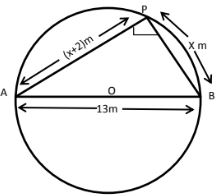Since, AB is a diameter and P is a point in the semi - circle ΔAPB is right angled at P.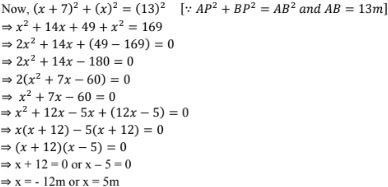Since, BP cannot be in negative value (or) distances cannot be negative values,
The required values of BP and AP are 5m and 12 m respectively.
∴ The pole has to be erected at a distance 5 meters from the gate B.

9. In a class test, the sum of the marks obtained by P in Mathematics and science is 28. Had he got 3 marks more  in Mathematics and 4 marks less in science. The product of his marks, would have been 180. Find his marks in the two subjects.

Solution

Let number of marks obtained by P in mathematics and science be x and y respectively.
Given that sum of these two is 28
⇒ x + y = 28
⇒ x = 28 - y
Given that if x becomes (x + 3) i.e. marks in mathematics is increased by 3 and y becomes (y - 4) i.e., marks in science is decreased by 4, The product of these two becomes by 4,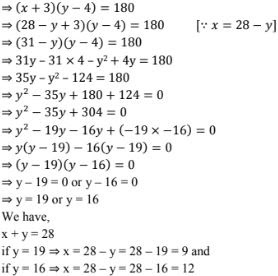∴ Marks in mathematics = 9 and Marks in Science = 19
or Marks in mathematics = 12 and Marks in Science  = 16

10. In a class test, the sum of Shefali's marks in Mathematics and English in 30. Had she got 2 marks more in Mathematics and 3 marks less in English, the product of her marks would have been 210. Find her marks in two subjects.

Solution

Let marks of shefali in Mathematics and English be x and y respectively.
Given that sum of these two is 30 ⇒ x + y = 30  ⇒ x = 30 - y
given that if x becomes (x + 2) i.e. marks in mathematics is increased by 2
and y becomes (y - 3) i.e. marks in English is decreased by 3.
the product at these two becomes 210∴ Marks in mathematics = 13 and marks in english = 17
or marks in mathematics = 12 and marks in english = 18

11. A cottage industry produces a certain number of pottery articles in a day. It was observed on a particular day that the cost of production of each article (in rupees) was 3 more than twice the number of articles produced on that day. If the total cost of production on that day was Rs. 90, find the number of articles produced and the cost of each article.

Solution

Let the number of articles produced on a particular day be x.
Total cost of production on that particular day = Rs. 90
Given ⇒ Cost of production of each article = (Total cost of production)/(no. of articles produced) = Rs.  90/x
But given that, the cost of production of each article was 3 more than twice the no. of article produced on that day i.e., Rs (2x + 3)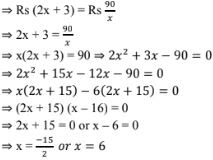Since, number of articles x cannot be a negative value, the required value of number of articles produced on a particular day x = 6.